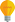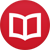# How to calculate the factorial of 1/2

by Jakub MarianTip: See my list of theMost Common Mistakes in English. It will teach you how to avoid mis­takes with com­mas, pre­pos­i­tions, ir­reg­u­lar verbs, and much more.

As I proved in my article on integration by parts (at the end), the factorial of a natural number $n$ can be calculated using the following integral:

$$n! = ∫_0^∞ x^ne^{-x}\,dx$$

The interesting thing about this integral is that it also works for $n$ that is not a natural number (and the result is a nice smooth function of $n$). The factorial of one half ($0.5$) is thus defined as

$$(1/2)! = ∫_0^∞ x^{1/2}e^{-x}\,dx$$

We will show that:

$$(1/2)! = \frac{√\pi}2$$

How to go about calculating the integral? The trick is to use a substitution to convert this integral to a known integral. First, we use integration by parts once, which will give us a form that is easier to work with:

$$∫_0^∞ \overbrace{x^{1/2}}^u\overbrace{e^{-x}}^{v’}\,dx = \l[\overbrace{x^{1/2}}^u\overbrace{(-e^{-x})}^v\r]_0^∞ - ∫_0^∞ \overbrace{\frac12 x^{-1/2}}^{u’}\overbrace{(-e^{-x})}^v\,dx$$

The term in the brackets is $0$. Let’s apply the substitution $y = x^{1/2}$ (i.e. $dy = \frac12 x^{-1/2}dx$) to the second integral (with the negative sign taken out):

$$∫_0^∞ \frac12 x^{-1/2}e^{-x}\,dx = ∫_0^∞ e^{-y^2}\,dy\,.$$

Since $e^{-y^2}$ is an even function, it is easy to see that

$$∫_0^∞ e^{-y^2}\,dy = ∫_{-∞}^0 e^{-y^2}\,dy = \frac12 ∫_{-∞}^∞ e^{-y^2}\,dy$$

(the function $e^{-y^2}$ looks the same “to the right of $0$” as “to the left of $0$”). The rightmost integral is known as the Gaussian integral, and I have shown a way to solve it in a separate article. The value turns out to be $√\pi$, so we get:

$$(1/2)! = \frac12 ∫_{-∞}^∞ e^{-y^2}\,dy = \frac{√\pi}{2}\,.$$

By the way, I have written several educational ebooks. If you get a copy, you can learn new things and support this website at the same time—why don’t you check them out?